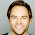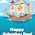Thursday, June 5, 2014

The Matrix, Inverse

For matrices there is no such thing as division, you can multiply but can’t divide.  Multiplying by the inverse matrix results in the same thing.    The inverse of a matrix A is A-1 only when:  A × A-1=  A-1 × A = I.   In other words multiplying a matrix by its inverse equals the identity matrix.

Finding the inverse of a 2x2 matrix is simple; there is a formula for that.  The bigger the matrix the bigger the problem.   There are two methods to find the inverse of a matrix: using minors or using elementary row operations (also called the Gauss-Jordan method), both methods are equally tedious.   We’ll be using the latter to find the inverse of matrices of order 3x3 or larger.   For a review of matrix elementary row operations click here.

This is one of the things you really want Symbolab to do the magic.

I neglected to mention that the formula is using the determinant, Symbolab does that too:

Let’s zoom into the row operations:

…told you it’s no fun

Here’s the link to an example with a 4x4 matrix, not for the weakhearted - click here.

Cheers,
Michal

1.I have been dotty by reading your blog because it has a unique data.
epistane

2.Check out some dissertation acknowledgements examples right now, so it will be easier for you to accomplish such task in the future!

3.this questions is very difficult. but i guess its solved now.
slashdot
choice of games
call of war
change
foro inu

4.matrix is complicated theory.
patch
pacman
scoop
cyber lord
Elster
lambgoat

5.6.7.step by step solve this questions.
scoop
zagl
8.9.